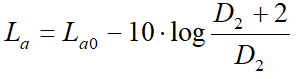# Aerodynamic Noise: IEC Standard - Intergraph Smart Instrumentation - 13.1 - Reference - Hexagon PPM

## Integraph Smart Instrumentation Sizing Equations

Language
English (United States)
Product
Intergraph Smart Instrumentation
Search by Category
Reference
Smart Instrumentation Version
13.1

*D2 [m], d [m], P1 [Pa-a], P2 [Pa-a], W[kg/s]

*FL=FLP/Fp

1. Calculate the downstream parameters: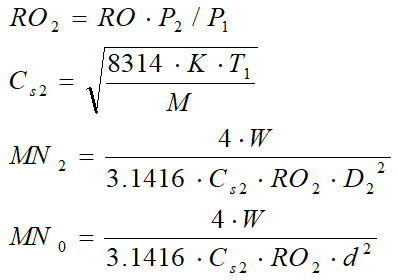2. Calculate the following pressures: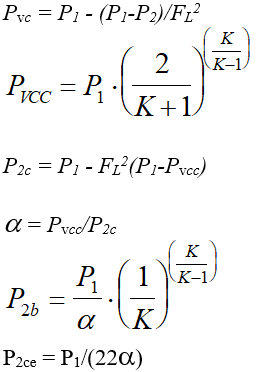3. Calculate Fd,Dj, Mj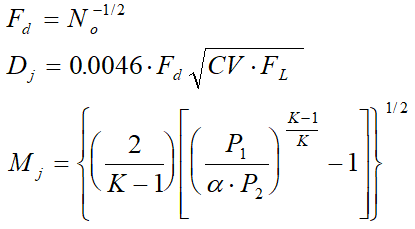4. Now 5 regimes are possible:

1. Regime I - P1 > P2 > P2c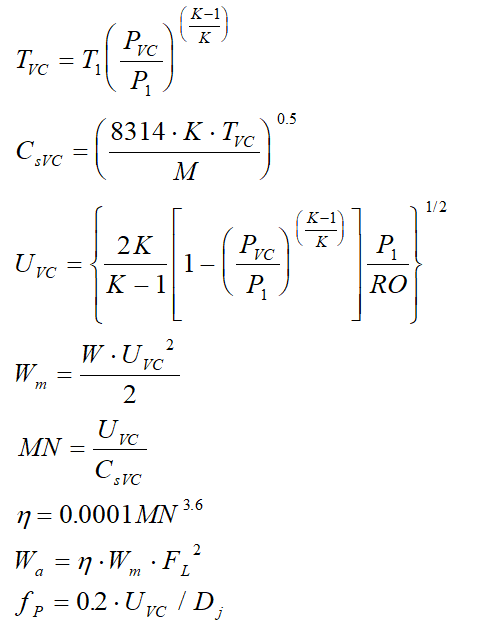2. Common calculations for II - V Regimes: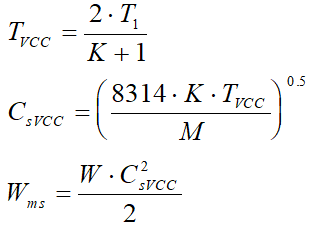3. Regime II - P2c > P2 > Pncc: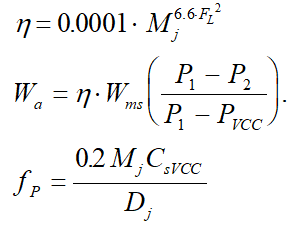4. Regime III - Pncc > P2 > P2b: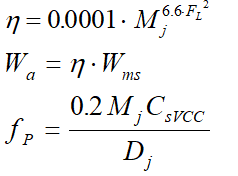5. Regime IV - P2b > P2 > P2ce: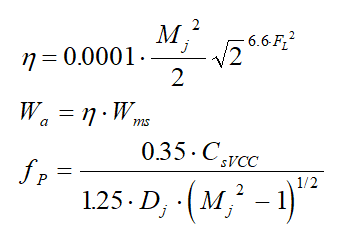6. Regime V - P2ce > P2 > 0: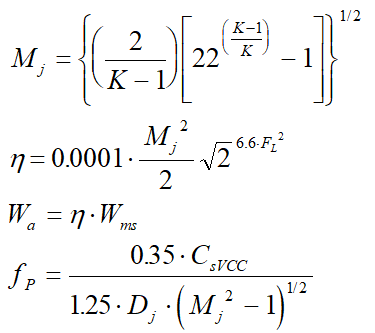5. Calculate Lpi: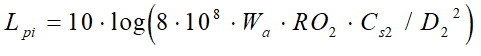6. Calculate TLƒr: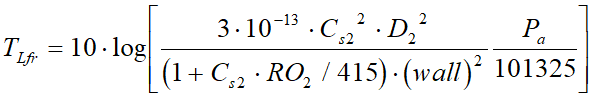7. Calculate ƒr and ƒo: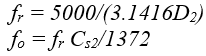8. Three cases are possible when calculating DTLfp:

1.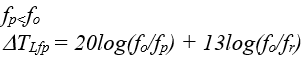2.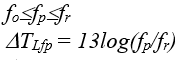3.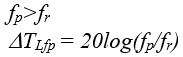9. Calculate transmission lost: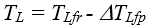10. Calculate Lg: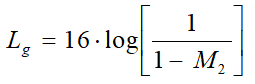11. Calculate sound level at the outside diameter of the pipe: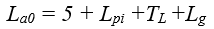12. Calculate sound level at a distance of 1m from the pipe wall: